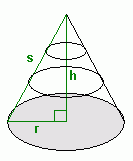From: Mirnela, a secondary school student

Hi, I'm trying to figure out a problem for homework and I don't understand how to any of this so if you can place help me I would really appreciate it!

The direction is to find the surface area of the cone. Now I have the cone drawn in my book. I know all the formulas and everything but I just don't understand how to find the slant height of this cone. The height is 40 and the radius is 9. How do I find the slant height of this??

Oh and also I have a formula when trying to find the volume of a cone: V=B(the area of the base) X h. Now I need to find a volume of a cone with a radius of 7 and height 45. How do you get the B?

Thank you so much for your help!

Hi Mirnela,

I've drawn a diagram to help out:Can you see from the diagram that the vertical height h must be perpendicular to the radius r of the base? That should tell you that the triangle in green is a right triangle, so you can use Pythagorus' Theorem to figure out the slant length s. In your second question, you asked for the area of the base of the cone. Can you see from the diagram (you have to visualize it in 3-D of course!) that the bottom of a cone is just a circle? To get the area B of a circle whose radius is r, you use the formula πr2.

Hope this helps!
Stephen La Rocque >